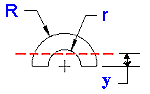Area Moment of Inertia Section Properties Half Tube Calculator

Area Moment of Inertia Section Properties: Half Tube Calculator

Area Moment of Inertia Section Properties of Half Tube Feature Calculator and Equations. This engineering calculator will determine the section modulus for the given cross-section. This engineering data is often used in the design of structural beams or structural flexural members.

 Description Equation Area Moment of Inertia Section Properties = ISection Modulus = Z = I/y I/y Radius of Gyration = Garea =ADistance to neutral axis = yVariables Inputs Inches mm "R" (Outside Radius) = "r" (Inside Radius) = Calculated Properties Inches mm Area Moment of Inertia I (Units4) = Section Modulus Z (Units3) = Radius of Gyration G (Unit) = Area A (Unit2) = Distance to Neutral Axis y = (unit)Membership Register | LoginHomeEngineering Book StoreEngineering ForumExcel App. DownloadsOnline Books & ManualsEngineering NewsEngineering VideosEngineering CalculatorsEngineering ToolboxGD&T Training Geometric Dimensioning TolerancingDFM DFA TrainingTraining Online EngineeringAdvertising CenterCopyright Notice